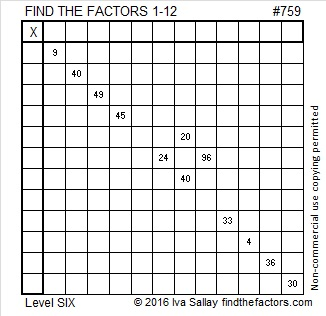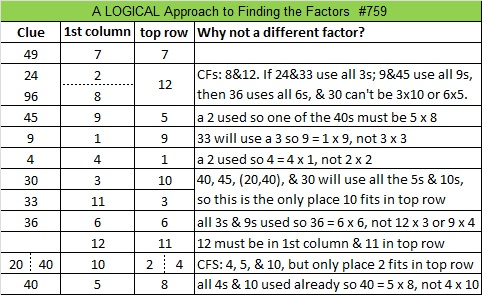# 759 and Level 6

• 759 is a composite number.
• Prime factorization: 759 = 3 x 11 x 23
• The exponents in the prime factorization are 1, 1, and 1. Adding one to each and multiplying we get (1 + 1)(1 + 1)(1 + 1) = 2 x 2 x 2 = 8. Therefore 759 has exactly 8 factors.
• Factors of 759: 1, 3, 11, 23, 33, 69, 253, 759
• Factor pairs: 759 = 1 x 759, 3 x 253, 11 x 69, or 23 x 33
• 759 has no square factors that allow its square root to be simplified. √759 ≈ 27.5499546.Here’s today’s puzzle. A logical way to find its solution is at the end of the post:Print the puzzles or type the solution on this excel file: 12 Factors 2016-01-25

—————————————

What else can I say about the number 759?

759 can be written as the sum of consecutive numbers seven different ways:

• 379 + 380 = 759; that’s 2 consecutive numbers.
• 252 + 253 + 254 = 759; that’s 3 consecutive numbers.
• 124 + 125 + 126 + 127 + 128 + 129 = 759; that’s 6 consecutive numbers.
• 64 + 65 + 66 + 67 + 68 + 69 + 70 + 71 + 72 + 73 + 74 = 759; that’s 11 consecutive numbers.
• 24 + 25 + 26 + 27 + 28 + 29 + 30 + 31 + 32 + 33 + 34 + 35 + 36 + 37 + 38 + 39 + 40 + 41 + 42 + 43 + 44 + 45  = 759; that’s 22 consecutive numbers.
• 22 + 23 + 24 + 25 + 26 + 27 + 28 + 29 + 30 + 31 + 32 + 33 + 34 + 35 + 36 + 37 + 38 + 39 + 40 + 41 + 42 + 43 + 44  = 759; that’s 23 consecutive numbers.
• 7 + 8 + 9 + 10 + 11 + 12 + 13 + 14 + 15 + 16 + 17 + 18 + 19 + 20 + 21 + 22 + 23 + 24 + 25 + 26 + 27 + 28 + 29 + 30 + 31 + 32 + 33 + 34 + 35 + 36 + 37 + 38 + 39 = 759; that’s 33 consecutive numbers.

759 is also the sum of five consecutive prime numbers: 139 + 149 + 151 + 157 + 163 = 759.

759 is a palindrome in two bases:

• 3C3 BASE 14 (C is 12 base 10)
• NN BASE 32 (N is 23 base 10)

—————————————This site uses Akismet to reduce spam. Learn how your comment data is processed.